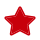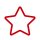# 去重

#### 语法格式

```SELECT [column_list]
FROM (
SELECT [column_list],
ROW_NUMBER() OVER ([PARTITION BY col1[, col2...]]
ORDER BY time_attr [asc|desc]) AS rownum
FROM table_name)
WHERE rownum = 1```

#### 语法说明

• ROW_NUMBER(): 从第一行开始，依次为每一行分配一个唯一且连续的号码。
• PARTITION BY col1[, col2...]: 指定分区的列，例如去重的键。
• ORDER BY time_attr [asc|desc]: 指定排序的列。所制定的列必须为时间属性。目前仅支持proctime。升序（ ASC ）排列指只保留第一行，而降序排列（ DESC ）则指保留最后一行。
• WHERE rownum = 1: Flink 需要 rownum = 1 以确定该查询是否为去重查询。

#### 示例

```SELECT order_id, user, product, number
FROM (
SELECT *,
ROW_NUMBER() OVER (PARTITION BY order_id ORDER BY proctime ASC) as row_num
FROM Orders)
WHERE row_num = 1;```

# 相关产品